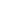U I 设计
IT 学院

# 新手入门！68个Python内置函数建议收藏学习

1. 数据类型

bool : 布尔型(True,False)

int : 整型(整数)

float : 浮点型(小数)

complex : 复数

2. 进制转换

bin() 将给的参数转换成二进制

otc() 将给的参数转换成八进制

hex() 将给的参数转换成十六进制

print(bin(10))  # 二进制:0b1010

print(hex(10))  # 十六进制:0xa

print(oct(10))  # 八进制:0o12

3. 数学运算

abs() 返回绝对值

divmode() 返回商和余数

round() 四舍五入

pow(a, b) 求a的b次幂, 如果有三个参数. 则求完次幂后对第三个数取余

sum() 求和

min() 求最小值

max() 求最大值

print(abs(-2))  # 绝对值:2

print(divmod(20,3)) # 求商和余数:(6,2)

print(round(4.50))   # 五舍六入:4

print(round(4.51))   #5

print(pow(10,2,3))  # 如果给了第三个参数. 表示最后取余:1

print(sum([1,2,3,4,5,6,7,8,9,10]))  # 求和:55

print(min(5,3,9,12,7,2))  #求最小值:2

print(max(7,3,15,9,4,13))  #求最大值:15

1. 序列

（1）列表和元组

list() 将一个可迭代对象转换成列表

tuple() 将一个可迭代对象转换成元组

print(list((1,2,3,4,5,6)))  #[1, 2, 3, 4, 5, 6]

print(tuple([1,2,3,4,5,6]))  #(1, 2, 3, 4, 5, 6)

（2）相关内置函数

reversed() 将一个序列翻转, 返回翻转序列的迭代器

slice() 列表的切片

lst = "你好啊"

it = reversed(lst)   # 不会改变原列表. 返回一个迭代器, 设计上的一个规则  print(list(it))  #['啊', '好', '你']

lst = [1, 2, 3, 4, 5, 6, 7]

print(lst[1:3:1])  #[2,3]

s = slice(1, 3, 1)  #  切片用的

print(lst[s])  #[2,3]

（3）字符串

str() 将数据转化成字符串

print(str(123)+'456')  #123456

format() 与具体数据相关, 用于计算各种小数, 精算等.

s = "hello world!"

print(format(s, "^20"))  #剧中

print(format(s, "<20"))  #左对齐

print(format(s, ">20"))  #右对齐

#     hello world!      # hello world!          #         hello world!  print(format(3, 'b' ))    # 二进制:11

print(format(97, 'c' ))   # 转换成unicode字符:a

print(format(11, 'd' ))   # ?进制:11

print(format(11, 'o' ))   # 八进制:13

print(format(11, 'x' ))   # 十六进制(?写字母):b

print(format(11, 'X' ))   # 十六进制(大写字母):B

print(format(11, 'n' ))   # 和d?样:11

print(format(11))         # 和d?样:11

print(format(123456789, 'e' ))      # 科学计数法. 默认保留6位小数:1.234568e+08

print(format(123456789, '0.2e' ))   # 科学计数法. 保留2位小数(小写):1.23e+08

print(format(123456789, '0.2E' ))   # 科学计数法. 保留2位小数(大写):1.23E+08

print(format(1.23456789, 'f' ))     # 小数点计数法. 保留6位小数:1.234568

print(format(1.23456789, '0.2f' ))  # 小数点计数法. 保留2位小数:1.23

print(format(1.23456789, '0.10f'))  # 小数点计数法. 保留10位小数:1.2345678900

print(format(1.23456789e+3, 'F'))   # 小数点计数法. 很大的时候输出INF:1234.567890

bytes() 把字符串转化成bytes类型

bs = bytes("今天吃饭了吗", encoding="utf-8")

bytearray() 返回一个新字节数组. 这个数字的元素是可变的, 并且每个元素的值得范围是[0,256)

ret = bytearray("alex" ,encoding ='utf-8')

print(ret)  #97

print(ret)  #bytearray(b'alex')

ret = 65  #把65的位置A赋值给ret

print(str(ret))  #bytearray(b'Alex')

ord() 输入字符找带字符编码的位置

chr() 输入位置数字找出对应的字符

ascii() 是ascii码中的返回该值 不是就返回u

print(ord('a'))  # 字母a在编码表中的码位:97

print(ord('中'))  # '中'字在编码表中的位置:20013

print(chr(65))  # 已知码位,求字符是什么:A

print(chr(19999))  #丟

for i in range(65536):  #打印出0到65535的字符

print(chr(i), end=" ")

print(ascii("@"))  #'@'

repr() 返回一个对象的string形式

s = "今天\n吃了%s顿\t饭" % 3

print(s)#今天# 吃了3顿    饭

print(repr(s))   # 原样输出,过滤掉转义字符 \n \t \r 不管百分号%

#'今天\n吃了3顿\t饭'

2. 数据集合

frozenset() 创建一个冻结的集合，冻结的集合不能进行添加和删除操作。

3. 相关内置函数

len() 返回一个对象中的元素的个数

sorted() 对可迭代对象进行排序操作 (lamda)

Iterable: 可迭代对象

key: 排序规则(排序函数), 在sorted内部会将可迭代对象中的每一个元素传递给这个函数的参数. 根据函数运算的结果进行排序

reverse: 是否是倒叙. True: 倒叙, False: 正序

lst = [5,7,6,12,1,13,9,18,5]

lst.sort()  # sort是list里面的一个方法

print(lst)  #[1, 5, 5, 6, 7, 9, 12, 13, 18]

ll = sorted(lst) # 内置函数. 返回给你一个新列表  新列表是被排序的  print(ll)  #[1, 5, 5, 6, 7, 9, 12, 13, 18]

l2 = sorted(lst,reverse=True)  #倒序

print(l2)  #[18, 13, 12, 9, 7, 6, 5, 5, 1]

#根据字符串长度给列表排序

lst = ['one', 'two', 'three', 'four', 'five', 'six']

def f(s):      return len(s)

l1 = sorted(lst, key=f, )  print(l1)  #['one', 'two', 'six', 'four', 'five', 'three']

enumerate() 获取集合的枚举对象

lst = ['one','two','three','four','five']

for index, el in enumerate(lst,1):    # 把索引和元素一起获取,索引默认从0开始. 可以更改

print(index)

print(el)

# 1

# one

# 2

# two

# 3

# three

# 4

# four

# 5

# five

all() 可迭代对象中全部是True, 结果才是True

any() 可迭代对象中有一个是True, 结果就是True

print(all([1,'hello',True,9]))  #True

print(any([0,0,0,False,1,'good']))  #True

zip() 函数用于将可迭代的对象作为参数, 将对象中对应的元素打包成一个元组, 然后返回由这些元组组成的列表. 如果各个迭代器的元素个数不一致, 则返回列表长度与最短的对象相同

lst1 = [1, 2, 3, 4, 5, 6]

lst2 = ['醉乡民谣', '驴得水', '放牛班的春天', '美丽人生', '辩护人', '被嫌弃的松子的一生']

lst3 = ['美国', '中国', '法国', '意大利', '韩国', '日本']

print(zip(lst1, lst1, lst3))  #<zip object at 0x00000256CA6C7A88>

for el in zip(lst1, lst2, lst3):

print(el)

# (1, '醉乡民谣', '美国')

# (2, '驴得水', '中国')

# (3, '放牛班的春天', '法国')

# (4, '美丽人生', '意大利')

# (5, '辩护人', '韩国')

# (6, '被嫌弃的松子的一生', '日本')

fiter() 过滤 (lamda)

function: 用来筛选的函数. 在?lter中会自动的把iterable中的元素传递给function. 然后根据function返回的True或者False来判断是否保留留此项数据 , Iterable: 可迭代对象

def func(i):    # 判断奇数

return i % 2 == 1

lst = [1,2,3,4,5,6,7,8,9]

l1 = filter(func, lst)  #l1是迭代器

print(l1)  #<filter object at 0x000001CE3CA98AC8>

print(list(l1))  #[1, 3, 5, 7, 9]

map() 会根据提供的函数对指定序列列做映射(lamda)

def f(i):

return i

lst = [1,2,3,4,5,6,7,]

it = map(f, lst) # 把可迭代对象中的每一个元素传递给前面的函数进行处理. 处理的结果会返回成迭代器print(list(it))  #[1, 2, 3, 4, 5, 6, 7]

locals() 返回当前作用域中的名字

globals() 返回全局作用域中的名字

def func():

a = 10

print(locals())  # 当前作用域中的内容

print(globals())  # 全局作用域中的内容

print("今天内容很多")

func()  # {'a': 10}

# {'__name__': '__main__', '__doc__': None, '__package__': None, '__loader__':

# '__spec__': None, '__annotations__': {}, '__builtins__': <module 'builtins'

# (built-in)>, '__file__': 'D:/pycharm/练习/week03/new14.py', '__cached__': None,

#  'func': <function func at 0x0000026F8D6B97B8>}

# 今天内容很多

range() 生成数据

next() 迭代器向下执行一次, 内部实际使用了__ next__()方法返回迭代器的下一个项目

iter() 获取迭代器, 内部实际使用的是__ iter__()方法来获取迭代器

for i in range(15,-1,-5):

print(i)

# 15

# 10

# 5

# 0

lst = [1,2,3,4,5]

it = iter(lst)  #  __iter__()获得迭代器  print(it.__next__())  #1

print(next(it)) #2  __next__()

print(next(it))  #3

print(next(it))  #4

eval() 执行字符串类型的代码. 并返回最终结果

exec() 执行字符串类型的代码

compile() 将字符串类型的代码编码. 代码对象能够通过exec语句来执行或者eval()进行求值

s1 = input("请输入a+b:")  #输入:8+9

print(eval(s1))  # 17 可以动态的执行代码. 代码必须有返回值

s2 = "for i in range(5): print(i)"

a = exec(s2) # exec 执行代码不返回任何内容  # 0

# 1

# 2

# 3

# 4

print(a)  #None

# 动态执行代码  exec("""

def func():

print(" 我是周杰伦")

""" )

func()  #我是周杰伦  code1 = "for i in range(3): print(i)"

com = compile(code1, "", mode="exec")   # compile并不会执行你的代码.只是编译

exec(com)   # 执行编译的结果  # 0

# 1

# 2

code2 = "5+6+7"

com2 = compile(code2, "", mode="eval")

print(eval(com2))  # 18

code3 = "name = input('请输入你的名字:')"  #输入:hello

com3 = compile(code3, "", mode="single")

exec(com3)  print(name)  #hello

print() : 打印输出

input() : 获取用户输出的内容

print("hello", "world", sep="*", end="@") # sep:打印出的内容用什么连接,end:以什么为结尾

#hello*world@

hash() : 获取到对象的哈希值(int, str, bool, tuple). hash算法:(1) 目的是唯一性 (2) dict 查找效率非常高, hash表.用空间换的时间 比较耗费内存

s = 'alex'

print(hash(s))  #-168324845050430382

lst = [1, 2, 3, 4, 5]

print(hash(lst))  #报错,列表是不可哈希的

id() :  获取到对象的内存地址  s = 'alex'

print(id(s))  #2278345368944

open() : 用于打开一个文件, 创建一个文件句柄

f = open('file',mode='r',encoding='utf-8')

f.close()

__ import__() : 用于动态加载类和函数

# 让用户输入一个要导入的模块

import os

name = input("请输入你要导入的模块:")

__import__(name)    # 可以动态导入模块

help() : 函数用于查看函数或模块用途的详细说明

print(help(str))  #查看字符串的用途

callable() : 用于检查一个对象是否是可调用的. 如果返回True, object有可能调用失败, 但如果返回False. 那调用绝对不会成功

a = 10

print(callable(a))  #False  变量a不能被调用

#  def f():      print("hello")

print(callable(f))   # True 函数是可以被调用的

dir() : 查看对象的内置属性, 访问的是对象中的__dir__()方法

print(dir(tuple))  #查看元组的方法

•2014期学员黄雨婷作品

•2103期学员沈继龙作品

•2105期学员秦洋洋作品

•2108期学员陆昶存作品

•2107期学员赵锦威作品

•2104期学员胡婷婷作品

•2107期学员何亮作品

•2103期学员张子安作品

• 作为一个企业，在互联网上建立自己的网站，最显而易见的目的就是可以向世界展示自己的企业风采，让更多人了解自己的企业，使企业能够在......

• 当下，有很多年轻人是通过网络来进行学习的，这在一定程度上，催生了很多网络教育平台。然而，随着教育平台如雨后春笋般冒了出来，在挑......

• 如今的PS，在我们的生活中可以说是无处不在了，作为专业的图像处理软件，PS不仅能让你更对已有的位图图像进行编辑加工处理以及运用......

• 学习编程是一件需要耐心和精力的事情，因为编程是一个需要在遇到问题的时候，提出猜想，进行调试，排除一些可能性的。那么，什么样的人......

• 编程是一个大热的行业，现在想学习编程的同学太多了，选择去哪里进行学习编程已经成为了很多同学所考虑的问题。目前市场上有很多学习编......

• Premiere提供了采集、剪辑、调色、美化音频、字幕添加、输出、DVD刻录的一整套流程，今天我们来聊一聊新手经常遇到的问题，......×